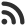Feed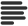Articles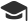Tutorials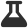Lab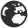CompaniesLeaderboard
 DG Student at BIT Mesra Oct. 12, 2020, 12:56 p.m. ⋅ 823 views

# TCS NQT aptitude questions set 8

Q131. Two pipes can fill the cistern in 10 hr and 20 hr respectively, while the third empty it in 30 hr. If all pipes are opened simultaneously, then the cistern will be filled in :

a) 7.5

b) 8.5

c) 8

d) None of these

Ans. (b)

Q132. Thomas takes 7 days to paint a house completely whereas Raj would require 9 days to paint the same house completely. How many days will take to paint the house if both them work together. (Give answers to the nearest integer) ?

a) 4

b) 2

c) 5

d) 3

Ans. (a)

Q133. How many liters of a 90% of concentrated acid needs to be mixed with a 75% solution of concentrated acid to get a 30 liter solution of 78% concentrated acid ?

a) 3

b) 8

c) 9

d) 6

Ans. (d)

Q134. Average marks of a,b,c is 48. When d joins average becomes 47. E has 3 more marks than d. Average marks of b,c,d,e is 48. What is the marks of a ?

a) 43

b) 42

c) 53

d) 56

Ans. (a)

Q135. A sum of money is borrowed and paid back in two annual installments of Rs.882 each allowing 5% C.I.The sum borrowed was :

a) Rs. 1680

b) Rs. 1142

c) Rs. 640

d) Rs. 1640

Ans. (d)

Q136. How many parallelograms are formed by a set of 4 parallel lines intersecting an other set of 7 parallel lines.

a) 125

b) 126

c) 127

d) 128

Ans. (b)

Q137. A completes 80% of a work in 20 days. Then B also joins and A and B together finish the remaining work in 3 days. How long does it need for B if he alone completes the work ?

a) 37.5

b) 32

c) 32.5

d) 37

Ans. (a)

Q138. 3 mangoes and 4 apples costs Rs 85. 5 apples and 6 peaches costs Rs. 122. 6 mangoes and 2 peaches cost Rs.114. what is the combined price of 1 apple, 1 peach and 1 mango ?

a) Rs 37

b) Rs 39

c) Rs 35

d) Rs 36

Ans. (a)

Q139. Find the probability that a leap year chosen at random will contain 53 Sundays.

a) 2/7

b) 3/7

c) 1/49

d) 1/7

Ans. (a)

Q140. What is the remainder of (32^31^301) when it is divided by 9 ?

a) 3

b) 5

c) 1

d) None of these

Ans. (b)

Q141. In how many ways can 2310 be expressed as product of three factors ?

a) 41

b) 56

c) 23

d) 46

Ans. (a)

Q142. How many lattice points are there between (2,0) and (16,203) ?

a) 8

b) 10

c) 14

d) 15

A. (a)

Q143. 10 people are there, they are shaking hands together, how many hand shakes possible, if they are in no pair of cyclic sequence.

a) 9

b) 12

c) 10

d) 45

Ans. (d)

Q144. A man sold 12 candies in 10\$ had loss of b% then again sold 12 candies at 12\$ had profit of b% find the value of b.

a) 9

b) 12

c) 10

d) 11

Ans. (d)

Q145. In how many ways can seven ‘#’ symbol and five ‘*’ symbol be arranged in a line so that no two ‘‘ symbols occur together ?

a) 44

b) 48

c) 28

d) 56

Ans. (d)

Q146. 1100 boys and 700 girls are examined in a test; 42% of the boys and 30% of the girls pass. Find the percentage of failed students.

a) 58%

b) 63%

c) 64%

d) 78%

Ans. (b)

Q147. If the numerator of a fraction be increased by 15% and its denominator be diminished by 8%, the value of fraction is 15/16. Find the original fraction.

a) 15/16

b) 3/2

c) 3/4

d) 3/7

Ans. (c)

Q148. Kush bought 40 pens at the marked price of 36 pens from a wholesaler. If he sells these pens giving a discount of 1%, what is profit percent ?

a) 5%

b) 15%

c) 20%

d) None of these

Ans. (d)

Q149. In how many different ways can the letters of the word ‘BANKING’ be arranged so that vowels always come together.

a) 240

b) 425

c) 540

d) 720

Ans. (d)

Q150. A cow and a horse are bought for Rs.200000. The cow is sold at a profit of 20% and the horse is sold at a loss of 10%. The overall gain is Rs. 4000.The cost pric of the cow is :

a) 130000

b) 80000

c) 70000

d) None of these

Ans. (b)

Q151. Divide 50 into two parts such that the sum of their reciprocals is 1/12, the numbers are :

a) 24, 26

b) 28, 22

c) 27, 23

d) 20, 30

Ans. (d)

Q152. In how many ways, can we distribute 10 pencils to 4 children so each child gets atleast one pencil ?

a) 9C3

b) 10C3

c) 14C4

d) 10C3

Ans. (a)

Q153. A motorist covers a distance of 39 km in 45 min by moving at a speed of x kmph for the first 15 min, then moving at double the speed for the next 20 min, and then again moving at his original speed for the rest of the journey. Find x.

a) 60

b) 48

c) 36

d) 45

Ans. (c)

Q154. If ACEFG+HECJHL=LCFHEJ then
L+C+F+H+E+J=?

a) 15

b) 10

c) 20

d) 30

Ans. (d)

Events

Nov. 28, 2018, 5:30 p.m.

Python from zero to hero

place Delhi

Aug. 13, 2018, 5:30 p.m.

Python from zero to hero

place Bangalore ( HackersFriend office BTM Layout)